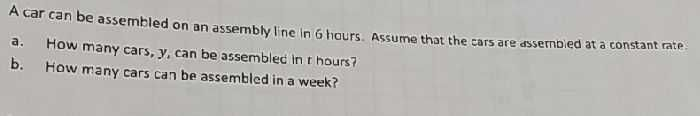### ¿Todavía tienes preguntas de matemáticas?

Pregunte a nuestros tutores expertos
Algebra
PreguntaA car can be assembled on an assembly line in $$6$$ hours. Assume that the cars are dssembied at a constant rate. a. How many cars, $$y$$ , can be assembled in t hours? b. How many cars can be assembled in a week?

a.$$y= \frac{t}{6}$$